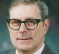## Non-zero DFT components where zero is expected?Started by 2 years ago6 replieslatest reply 2 years ago220 views

This question has been asked before in dsp.stackexchange.com but I am reposting it here because very few questions are answered on that site.

Edit: I got a few replies on dsp.stackexchange.com. But if you would like to add something to those answers, I would be happy to read and learn more :)

I am implementing DFT in Octave. Here's my code:

function [real_comp, imag_comp] = mydft (samples)
N = columns(samples);
for m = 1:N
real_accum = 0;
imag_accum = 0;
for n = 1:N
real_factor = cos((2 * pi * (n-1) * (m-1)) / N);
# imag_factor = -I * sin((2 * pi * (n-1) * (m-1)) / N);
imag_factor = sin((2 * pi * (n-1) * (m-1)) / N);
real_accum += real_factor * samples(n);
imag_accum += imag_factor * samples(n);
endfor
real_comp(m) = real_acuum;
imag_comp(m) = imag_accum;
endfor
endfunction


My goal is to compute the magnitude at each DFT bin and plot it. I am passing in a list of real-only samples and storing the real and imaginary components in different variables. Here's the sample vector that I am using:

samples = [0.3535, 0.3535, 0.6464, 1.0607, 0.3535, -1.0607, -1.3535, -0.3535]


The samples are carefully chosen to prevent spectral leakage at fs = 8KHz. The graph of the DFT magnitude is exactly what I expect. There's nothing wrong with that. But what I don't get is that some components in my 8 point DFT which are supposed to be zero, are not exactly zero but rather a very small, close-to-zero value. The built-in fft() function, on the other hand, has zeroed out components where they should be.

For example, I print the elements of output vector produced by my DFT and Octave's FFT using the code below:

fft_out = fft(samples);
[real, imag] = mydft(samples);
dft_out = real + (-I * imag);
for i=1:columns(samples)
disp(fft_out(i));
disp(dft_out(i));
printf("\n");
endfor


The point-to-point comparison is given in the table below:

Bin Index
FFT_OUT DFT_OUT
1 -1.0000e-04 -1.0000e-04
2 0.00000 - 3.99988i 4.7184e-16 - 3.9999e+00i
3 1.4141 + 1.4144i 1.4141 + 1.4144i
4 0.0000e+00 - 8.0820e-05i 1.3878e-16 - 8.0820e-05i
5 -1.0000e-04 -1.0000e-04 - 1.4350e-16i
6 0.0000e+00 + 8.0820e-05i 2.3037e-15 + 8.0820e-05i
7 1.4141 - 1.4144i 1.4141 - 1.4144i
8 0.00000 + 3.99988i -2.0817e-15 + 3.9999e+00i

Now, I wouldn't care too much about the tiny difference but if I compute the phase angles of fft_out and dft_out,

disp(arg(fft_out))
disp(arg(dft_out))


I get the sign flipped in some cases:

fft_out: 3.14159  -1.57080   0.78550  -1.57080   3.14159   1.57080  -0.78550   1.57080
dft_out:-3.14159  -1.57080   0.78550  -1.57080  -3.14159   1.57080  -0.78550   1.57080


So, is there something wrong with my DFT implementation? Is it just a rounding or precision issue?

[ - ]The only examples I see with sign reversals of phase are for n=1 and n=5, which are frequency bins n-1=0 and n-1=4. These are both, correctly, negative real numbers. The "phase" of a real number is 0 when the number is positive and pi when the number is negative. But -pi is the same as +pi when you are talking about a phase.

[ - ]It's just a precision issue.  For values smaller than +/-10^-10, substitute 0.0 and then feed it to your phase calculator.

Mantissa in double precision is 54 bits which is about 1/10^18.  So you are looking at 2 or 3 bits out of 54 which are noise.  It's doing what it's supposed to, but every calculation is not noise free so you have to ignore the noise.

You'll sometimes see "-0.0" printed on an output.  It's the same thing.  Just brute force it to exactly 0.0 and go from there.

[ - ]Hello bittersweet,

What you are observing is that the trigonometric functions are transcendental, which means that they need very very wide width representations of their sample values to approximate the actual values of their samples. None of this 4-decimal approximation stuff! The trig sequences with finite precision approximations fail to actually have zero valued projections between the basis sequences of the DFT.

The phase angle problem is related to where we place a cut on the unit circle. the cut at pi means +pi-epsi and -pi-epsi are 2pi apart rather that 2 epsi apart. Matlab has a function called unwrap that tries to suppress the phase jumps caused by the cut. Not always successful!

fred h

[ - ]edit to a previous post: I see you are inverting the imaginary to convert iDFT to DFT.

I think you should ask why fft is different from DFT (since you are not rounding in both cases).

FFT uses butterfly algorithm to speed up the computation at the expense of possibly some tiny "error". The DFT equation is slow, more accurate but it has more accumulated quantisation noise despite high fp resolution??

use this code and you see difference building up and also notice slow speed if (n) is large:

%%%%%%%%%%%%%%%%%%%%%%%%%%%%%%%%%%%%%%%%%%%%%%%%

%direct DFT versus fft

n = 2^10;

x = randn(1,n);

y = zeros(1,n);

for k = 1:n

for i = 1:n

f = exp(-1i*2*pi*(i-1)*(k-1)/n);

y(k) = y(k) + x(i)*f;

end

end

%compare to fft function

ref = fft(x,n);

plot(real(y) - real(ref));hold;

plot(imag(y) - imag(ref),'g-')

[ - ]WRT the rounding precision:  FFTs also use radix stages to perform the butterfly operations. These stages use simple addition/subtraction/swapping where possible rather than calculating the corresponding complex number and multiplying (save for twiddle factors). This is because it is considerably more efficient than using complex multiplication.  As a result, it could result in better accuracy for lower sample lengths. For example, if the FFT used a radix 8 calculation on your length 8 sequence, only four multiplies are required (by 0.707...), and only four output values would be effected.

The other comments here regarding precision are still valid, but that’s most likely what’s going on under the hood

[ - ]